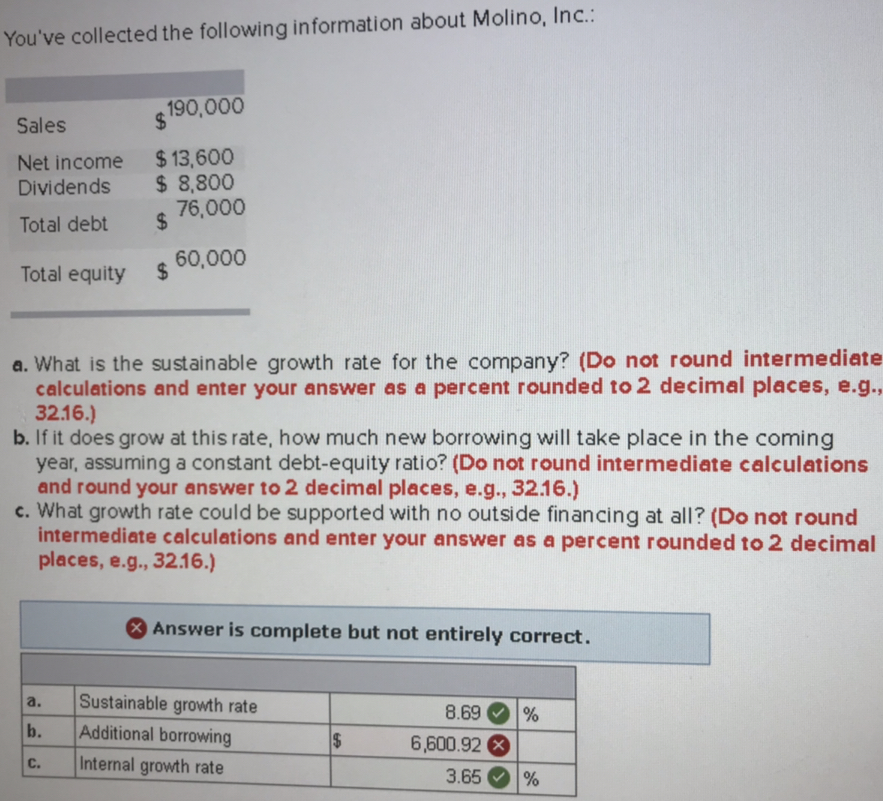# You've collected the following information about Molino, Inc. 190,000 Sales Net income $13,600 Dividends 8,800 bt$ 76,000 60,000 Total equity a. What is the sustainable growth rate for the company? (Do not round intermediate b. If it does grow at this rate, how much new borrowing will take place in the coming c. What growth rate could be supported with no outside financing at all? (Do not round calculations and enter your answer as a percent rounded to 2 decimal places, e.g, 3216.) year, assuming a constant debt-equity ratio? (Do not round intermediate calculations and round your answer to 2 decimal places, e.g., 32.16.) intermediate calculations and enter your answer as a percent rounded to 2 decimal places, e.g., 32.16.) Answer is complete but not entirely correct. a. Sustainable growth rate b. Additional borrowing c. Internal growth rate 8.69 )% 6,600.92 3.65 196

Question
2395 viewshelp_outlineImage TranscriptioncloseYou've collected the following information about Molino, Inc. 190,000 Sales Net income $13,600 Dividends 8,800 bt$ 76,000 60,000 Total equity a. What is the sustainable growth rate for the company? (Do not round intermediate b. If it does grow at this rate, how much new borrowing will take place in the coming c. What growth rate could be supported with no outside financing at all? (Do not round calculations and enter your answer as a percent rounded to 2 decimal places, e.g, 3216.) year, assuming a constant debt-equity ratio? (Do not round intermediate calculations and round your answer to 2 decimal places, e.g., 32.16.) intermediate calculations and enter your answer as a percent rounded to 2 decimal places, e.g., 32.16.) Answer is complete but not entirely correct. a. Sustainable growth rate b. Additional borrowing c. Internal growth rate 8.69 )% 6,600.92 3.65 196 fullscreen

1 Rating

### Want to see the step-by-step answer?